123456789，這幾個平常的數字其實並不簡單，每個數字的背後都內藏玄機，它也許是通往宇宙的鑰匙，甚至可能是人與神聯繫的密碼。我們今天就來探討一下3、6、9這三個數字的神奇之處。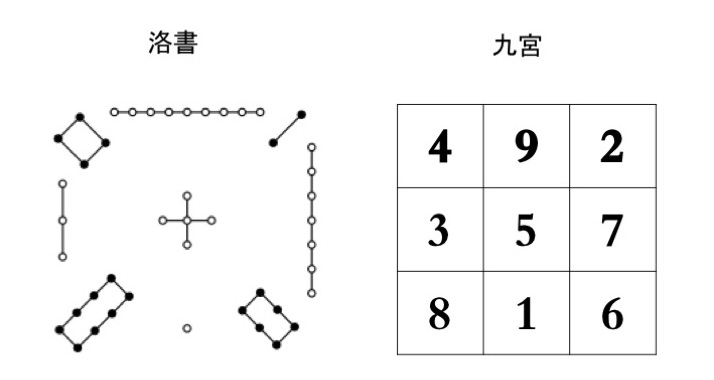#### 神奇一：9

1、從任何一排數字中（橫豎斜）取兩個任意組合的3位數相乘，其眾數和都是9。

492X294=144648，眾數和：1+4+4+6+4+8=27，2+7=9

951X591=562041，眾數和：5+6+2+0+4+1=18，1+8=9

456X465=212040，眾數和：2+1+2+0+4+0=9

2、任意兩排數字中，隨機三位數相乘，其結果的眾數和也都等於9。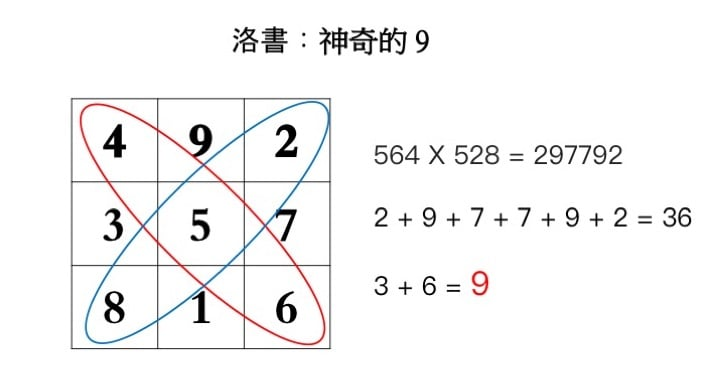3、任何一排中隨機三個三位數字相乘，其結果的眾數和同樣等於9。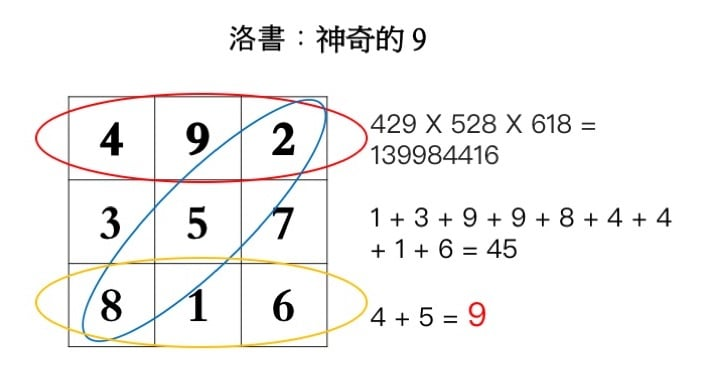4、任意三排的三組數字相加，其結果的眾數和為9。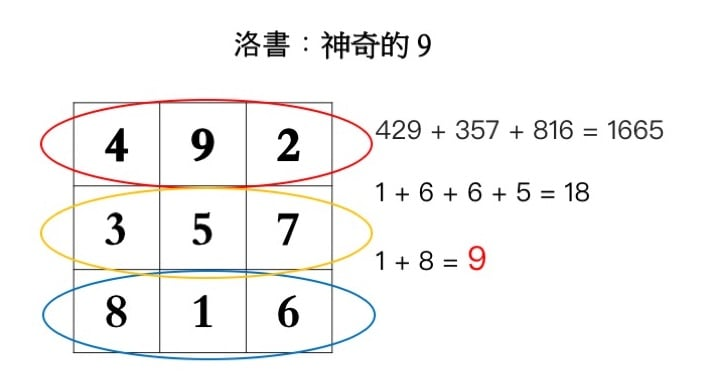#### 神奇二：6

4+9+2=15

4+5+6=15

4+3+8=15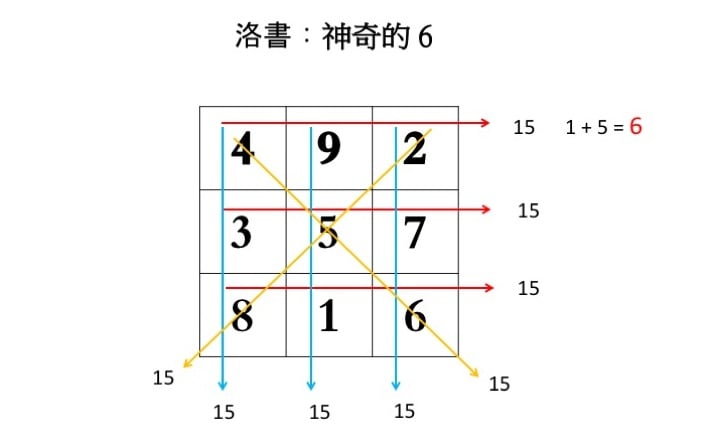#### 神奇三：3

294+672=966，9＋6＋6=21，2＋1=3

915＋735=1650，1＋6＋5=12，1+2=3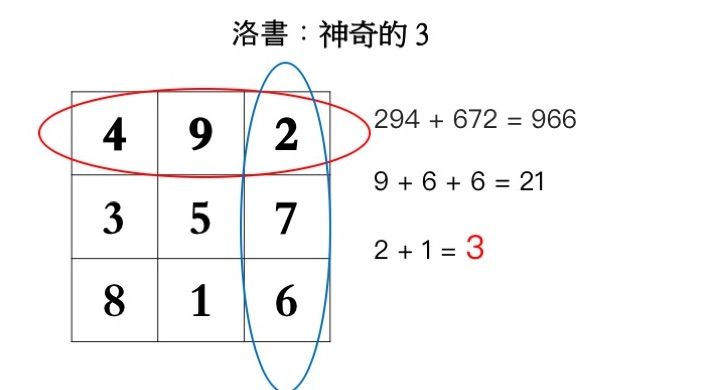#### 3、6、9特殊的存在

1 2 3 4 5 6 7 8 9 中，除了3、6、9外，任何兩位相鄰的數相加，其眾數和都等於3、6、9。例如：

1+2=3

2+4=6

4+5=9

5+7=12，1+2=3

7+8=15，1+5=6